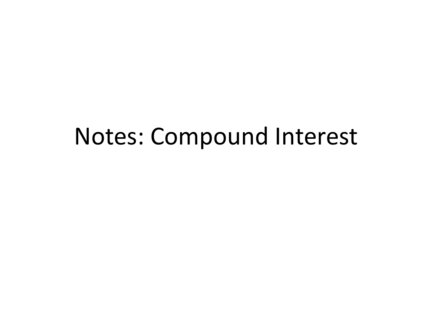# Compound InterestContributed by:This pdf includes the following topics:-
Compound interest
Principal
Compound interest formula
Examples
Notes
1. Notes: Compound Interest
2. A common application of exponential growth is
compound interest. Recall that simple interest
is earned or paid only on the principal.
Compound interest is interest earned or paid
on both the principal and previously earned
3.
For compound interest
• annually means “once per year” (n = 1).
• quarterly means “4 times per year” (n = 4).
• monthly means “12 times per year” (n = 12).
•Daily usually means “365 times per year”, or “366 times per year” during a leap
5. Ex 1: Write a compound interest function to model the situation.
Then find the balance after the given number of years.
\$1200 invested at a rate of 2% compounded quarterly; 3 years
Step 1 Write the compound
interest function for this
4(3)
situation.
0.02
= 1200 1 +
4 Step 2: Substitute 1200 for P, 0.02 for
= 1200 1 + 0.005 12 r, and 4 for n, 3 for t.
Simplify.
= 1200(1.005)12
Use a calculator and round to the
≈ 1274.01 nearest hundredth.
The balance after 3 years is \$1274.01.
6. Ex 2: Write a compound interest function to model the situation.
Then find the balance after the given number of years.
\$15,000 invested at a rate of 4.8% compounded monthly; 2 years
Step 1 Write the compound
interest function for this
12(2)
situation.
0.048
= 15000 1 +
12 Step 2: Substitute 1200 for P, 0.02 for
= 15000 1 + 0.004 24 r, and 4 for n, 3 for t.
Simplify.
= 15000(1.004)24
Use a calculator and round to the
≈ 16508.22 nearest hundredth.
The balance after 2 years is \$16,508.22.
7. Ex 3: Write a compound interest function to model the situation.
Then find the balance after the given number of years.
\$1200 invested at a rate of 3.5% compounded quarterly; 4 years
Step 1 Write the compound
interest function for this
4(4)
situation.
0.035
= 1200 1 +
4 Step 2: Substitute 1200 for P, 0.02 for
= 1200 1 + 0.00875 16 r, and 4 for n, 3 for t.
Simplify.
= 1200(1.000875)24
Use a calculator and round to the
≈ 1379.49 nearest hundredth.
The balance after 4 years is \$1379.49.
8. Ex 4: Write a compound interest function to model the situation.
Then find the balance after the given number of years.
\$4000 invested at a rate of 3% compounded monthly; 8 years
Step 1 Write the compound
interest function for this
12(8)
situation.
0.03
= 4000 1 +
12 Step 2: Substitute 1200 for P, 0.02 for
= 4000 1 + 0.0025 96 r, and 4 for n, 3 for t.
Simplify.
= 4000(1.0025)96
Use a calculator and round to the
≈ 5083.47 nearest hundredth.
The balance after 8 years is \$5083.47.
9. Ex 5: Write a compound interest function to model the situation.
Then find the balance after the given number of years.
\$4000 invested at a rate of 3% compounded monthly; 8 years
Step 1 Write the compound
interest function for this
12(8)
situation.
0.03
= 4000 1 +
12 Step 2: Substitute 1200 for P, 0.02 for
= 4000 1 + 0.0025 96 r, and 4 for n, 3 for t.
Simplify.
= 4000(1.0025)96
Use a calculator and round to the
≈ 5083.47 nearest hundredth.
The balance after 8 years is \$5083.47.